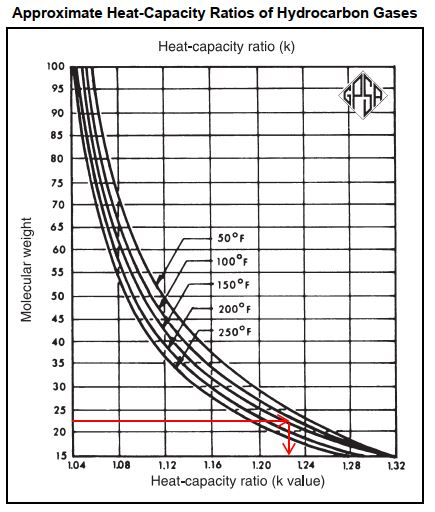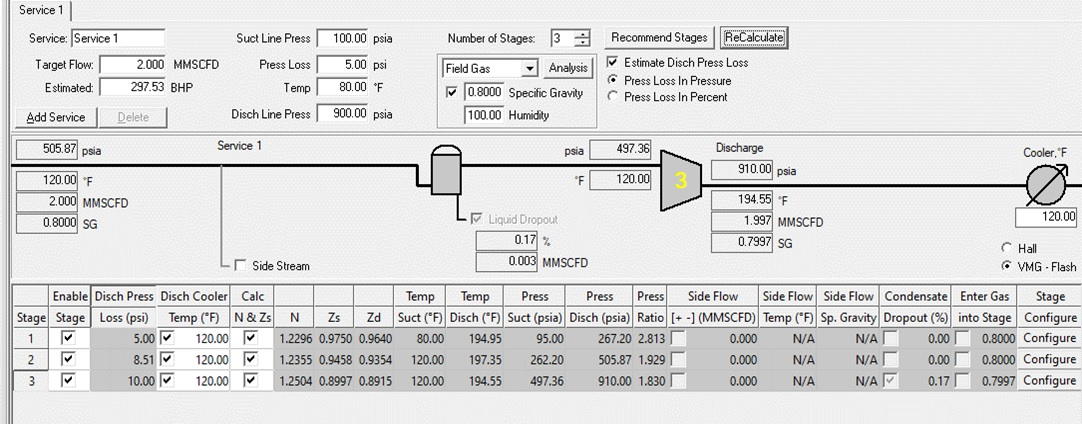# Reciprocating Compressor Power Calculation Part 2 (Three-Stage Compression)

In previous post, I shared how to calculate reciprocating compressor power if number of compression stage is two. In this post, I will use the same problem/example to estimate reciprocating compressor power if number of compression stage is 3. In the end of this posting series, I will show you the difference of each stage and to see how important to determine number of compression stages.

The method used to calculate reciprocating compressor is the same, whether it is two-stage, three-stage, or four-stage. There are several steps which is repetitive.

# Example

Compress 2 MMscfd of gas measured at 14.65 psia and 60oF. Intake pressure is 100 psia, and intake temperature is 100oF. Discharge pressure is 900 psia. The gas has a specific gravity of 0.80 (23 MW). What is the required horse power?

Assuming number of stages is 3

# Solution

## Step 1 – Calculate Overall Compression Ratio

Overall compression ratio is ratio of discharge pressure in absolute pressure and suction pressure in absolute pressure. So,

Pressure ratio = 900 psia/100 psia = 9

## Step 2 – Decide Number of Stage

Three compression stages.

## Step 3 – Determine Compression Ratio Per Stage

For three-stage compression, ratio per stage is = (overall compression ratio) ^ (1/3)

Ratio per stage = (9)^(1/3) = 2.08

## Step 4 – Determine 1st Stage Discharge Pressure

1st stage discharge pressure = compression ratio per stage x suction pressure

So, 1st stage discharge pressure = 2.08 x 100 psia = 208 psia

## Step 5 – Determine Suction Pressure for 2nd Stage

Assuming pressure drop = 5 psi,

Then 2nd stage suction pressure = 1st stage discharge pressure – pressure drop = 208 – 5 = 203 psia

## Step 6 – Determine Compression Ratio for 2nd Stage

As we considered pressure drop between 1st stage discharge pressure and 2nd stage suction pressure, compression ratio of 2nd stage is no longer the same as compression ratio of 1st stage.

For 3-stage compression, compression ratio for 2nd stage = (discharge pressure/2nd stage suction pressure) ^ (1/2)

Compression ratio for 2nd stage = (900/203) ^(1/2) = 2.11

## Step 7 – Determine 2nd Stage Discharge Pressure

2nd stage discharge pressure = compression ratio per at 2nd stage compression x suction pressure for 2nd stage

2nd stage discharge pressure = 2.11 x 203= 427 psi

## Step 8 – Determine k value (heat-capacity ratio)

K value or heat-capacity ratio is function of molecular weight and temperature. We will determine K value by curve. For most compression application, 150oF curve will be adequate. This should be checked after determining the average cylinder temperature.

So, for molecular weight 23 and temperature of 150oF, we get K value of 1.21.## Step 9 – Determine discharge temperature of 1st stage compression

Discharge temperature of compressor is function of compression ratio and K value. We can determine discharge temperature by chart. For compression ratio 2.08 (see Step 3) at first stage compression, K value of 1.21 (see Step 8), and suction temperature of 100oF, we get discharge temperature of 1st stage compression is 174oF.

Discharge temperature of compression can also be estimate by using the following equation.

By using equation above:

td at 1st stage compression = (100 + 460) x (2.08) ^ [(1.21-1)/1.21] – 460

(2.08 is compression ratio at first-stage compression)

td = 175.9oF

For easiness, we will use discharge temperature as per equation, which is 175.9oF

## Step 10 – Determine cylinder temperature of 1st stage compression

Cylinder temperature of 1st stage compression is average of suction temperature (100oF) and discharge temperature of 1st stage compression (175.9oC). Therefore, cylinder temperature of 1st stage compression is 137.95oF.

## Step 11 – Determine discharge temperature of 2nd stage compression

Step 11 is the same as Step 9. Assuming interstage cooling to 120oF.

For compression ratio 2.11 (see Step 6) at second stage compression, K value of 1.21, and suction temperature of 120oF, we get discharge temperature of 2nd stage compression is 196oF.

If we use equation as stated in Step 9, we can get discharge temperature:

td at 2nd stage compression = (120 + 460) x (2.11) ^ [(1.21-1)/1.21] – 460

(2.11 is compression ratio at second-stage compression)

td = 200oF

Discharge temperature we got from chart and from equation is quite similar. We will use discharge temperature from equation which is 200oF.

## Step 12 – Determine cylinder temperature of 2nd stage compression

Cylinder temperature of 2nd stage compression is average of suction temperature to 2nd stage compression (120oF) and discharge temperature of 2nd stage compression (200oC). Therefore, cylinder temperature of 1st stage compression is 160oF.

## Step 13 – Determine Compression Ratio for 3rd Stage

We considered there is pressure drop between 2nd stage discharge pressure and 3rd stage suction pressure. Therefore, compression ratio of 3rd stage may no longer the same as compression ratio of 1st stage or 2nd stage

For 3-stage compression, compression ratio for 3rd stage = (discharge pressure/3nd stage suction pressure)

3rd stage suction pressure = 2nd stage discharge pressure – pressure drop = 427.4 (see Step 7) – 5 = 422.4 psig

Compression ratio for 3nd stage = (900/422.4) = 2.13

## Step 14 – Determine Discharge Temperature of 3rd stage compression

If we use equation as stated in Step 9, we can get discharge temperature:

td at 3rd stage compression = (120 + 460) x (2.13) ^ [(1.21-1)/1.21] – 460

(2.08 is compression ratio at third-stage compression, and 120oF is suction temperature to 3rd stage compression which is equal to outlet cooler temperature)

td = 201oF

## Step 15 – Estimate compressibility factor (Z factor) at suction and discharge condition at each compression stage

From calculation above, we get:

1st stage (P suction = 100 psia, T suction = 100oF)

1st stage (P discharge = 208 psia, T discharge = 175.9 oF) ____ see Step 4 and Step 9

2nd stage (P suction = 203 psia, T suction = 120oF) ____ see Step 5. Assuming interstage cooling to 120oF

2nd stage (P discharge = 427 psia, T discharge = 201oF ____ (see step 7 and step 11)

3rd stage (P suction = 422 psia, T suction = 120oF) ____ see Step 5. Assuming interstage cooling to 120oF

3rd stage (P discharge = 900 psia, T discharge = 201oF ____ (see step 14)

Based on operating condition above, we will check compressibility factor based on charts. Please note that each chart is function of gas molecular weight. So, ensure you use proper chart.

In this example, molecular weight of gas is 23. So, I will use compressibility factor for gas with molecular weight 23.20.

1st stage, Z factor at suction condition = 0.98

1st stage, Z factor at discharge condition = 0.96

Average Z factor = (0.98 + 0.96)/2 = 0.97

Calculate the same thing for 2nd stage,

2nd stage, Z factor at suction condition = 0.95

2nd stage, Z factor at discharge condition = 0.935

Average Z factor = (0.95 + 0.935)/2 = 0.943

Calculate the same thing for 3rd stage,

3rd stage, Z factor at suction condition = 0.90

3rd stage, Z factor at discharge condition = 0.88

Average Z factor = (0.95 + 0.935)/2 = 0.89

## Step 16 – Estimate BHP per MMcfd from Chart

BHP per MMcfd is a function of compression ratio and K value. BHP per MMcfd read from figure below use a pressure base of 14.4 psia.

For compression ratio 2.09, and k value 1.21, we get BHP per MMcfd is 44.5 (1st stage)

For compression ratio 2.11, and k value 1.21, we get BHP per MMcfd is 45 (2nd stage)

For compression ratio 2.14, and k value 1.21, we get BHP per MMcfd is 44 (3rd stage)

## Step 17 – Check correction factor for low intake pressure and for specific gravity

Based on figure below, for ratio of compression 2.09; 2.11; and 2.14, correction factor for low intake pressure is 1.

Based on figure below, for ratio of compression 2.09; 2.11; and 2.08, correction factor for specific gravity is 1.

## Step 18 (Last Step) – Calculate BHP for each stage and total BHP

BHP = (BHP/MMcfd) x (PL/14.4) x (TS/TL) x (Z average) x (MMcfd)

PL is pressure base, which is 14.65 psia

Ts is suction temperature, which is 100oF (560oR)

TL is temperature base, which is 60oF (520oR)

For 1st stage, BHP is 44.5 x (14.65/14.4) x (560/520) x 0.97 x 2 = 94.58

For 2nd stage, T suction is 120oF (580oR)

Using the same equation, BHP is 45 x (14.65/14.4) x (580/520) x 0.943 x 2 = 96.26

For 3rd stage, T suction is 120oF (580oR)

Using the same equation, BHP is 44 x (14.65/14.4) x (580/520) x 0.89 x 2 = 88.87

Total BHP is 94.58 + 96.26 + 88.87 = 279.71

Based on calculation above, we can illustrate the results below.I also tried using Ariel Commercial to check if the result is similar. I found that the BHP is 297.53. The difference is about 6%.